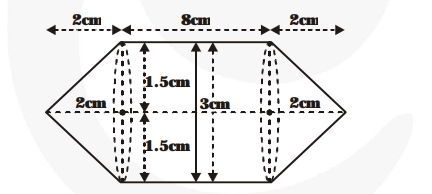# Rachel, an engineering student,Question:

Rachel, an engineering student, was asked to make a model shaped like a cylinder with two cones attached at its two ends by using a thin aluminium sheet. The diameter of the model is 3 cm and its length is 12 cm. If each cone has a height of 2 cm, find the volume of air contained in the model that Rachel made. (Assume the outer and inner dimensions of the model to be nearly the same).

Solution:

Volume of the cylindrical part

$=\pi \times(1.5)^{2} \times 8 \mathrm{~cm}^{3}=18 \pi \mathrm{cm}^{3}$

Volume of each conical part$=\frac{\mathbf{1}}{\mathbf{3}} \pi \times(1.5)^{2} \times 2 \mathrm{~cm}^{3}=\frac{\mathbf{3}}{\mathbf{2}} \pi \mathrm{cm}^{3}$

Therefore, the volume of the air = The volume of cylindrical part + The volumes of two conical parts

$=18 \pi+2 \times \frac{\mathbf{3}}{\mathbf{2}} \pi \mathrm{cm}^{3}=21 \pi \mathrm{cm}^{3}$

$=21 \times \frac{\text { 22 }}{\text { 7 }} \mathrm{cm}^{3}=66 \mathrm{~cm}^{3}$